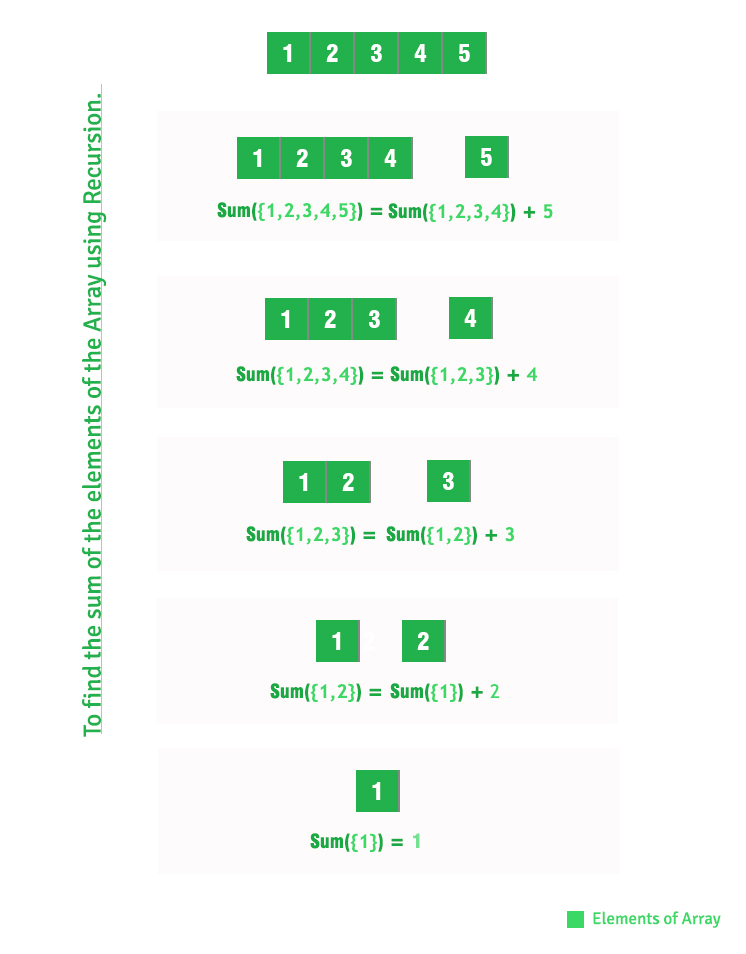Sum of array elements using recursion

• Difficulty Level : Basic
• Last Updated : 23 Jun, 2021

Given an array of integers, find sum of array elements using recursion.
Examples:

Input : A[] = {1, 2, 3}
Output : 6
1 + 2 + 3 = 6

Input : A[] = {15, 12, 13, 10}
Output : 50

Recommended: Please solve it on “PRACTICE” first, before moving on to the solution.

We have discussed iterative solution in below post.
Sum of elements in a given array
In this post, recursive solution is discussed.

C++

 // C++ program to find sum of array// elements using recursion.#include  // Return sum of elements in A[0..N-1]// using recursion.int findSum(int A[], int N){    if (N <= 0)        return 0;    return (findSum(A, N - 1) + A[N - 1]);} // Driver codeint main(){    int A[] = { 1, 2, 3, 4, 5 };    int N = sizeof(A) / sizeof(A);    printf("%dn", findSum(A, N));    return 0;}

Java

 // Java program to find sum of array// elements using recursion. class Test {    static int arr[] = { 1, 2, 3, 4, 5 };     // Return sum of elements in A[0..N-1]    // using recursion.    static int findSum(int A[], int N)    {        if (N <= 0)            return 0;        return (findSum(A, N - 1) + A[N - 1]);    }     // Driver method    public static void main(String[] args)    {        System.out.println(findSum(arr, arr.length));    }}

Python

 # Python program to find sum of array# elements using recursion.  # Return sum of elements in A[0..N-1] # using recursion.  def _findSum(arr, N):     if len(arr)== 1:        return arr     else:        return arr+_findSum(arr[1:], N) # driver codearr =[]# input values to listarr = [1, 2, 3, 4, 5]  # calculating length of arrayN = len(arr)  ans =_findSum(arr,N)print (ans)  # This code is contributed by Khare Ishita

C#

 // C# program to find sum of array// elements using recursion.using System; class Test {         static int []arr = {1, 2, 3, 4, 5};     // Return sum of elements in    // A[0..N-1] using recursion.    static int findSum(int []A, int N)    {        if (N <= 0)            return 0;        return (findSum(A, N - 1) + A[N - 1]);    }     // Driver Code    public static void Main()    {        Console.Write(findSum(arr, arr.Length));    }} // This code is contributed by Nitin Mittal.



Javascript



Output:

15

How does above recursive solution work?This article is contributed by Prakhar Agrawal. If you like GeeksforGeeks and would like to contribute, you can also write an article using write.geeksforgeeks.org or mail your article to review-team@geeksforgeeks.org. See your article appearing on the GeeksforGeeks main page and help other Geeks.